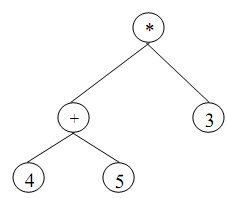## Algorithm for inorder traversals, Data Structure & Algorithms

Assignment Help:

Step-1: For the current node, verify whether it contain a left child. If it has, then go to step-2 or else go to step-3

Step-2: Repeat step-1 for left child

Step-3: Visit (that means printing the node in our case) the current node

Step-4: For the current node verify whether it contain a right child. If it contain, then go to step-5

Step-5: Repeat step-1 for right childFigure: A binary tree

The preoreder & postorder traversals are similar to that of a general binary tree. The general thing we have seen in all of these tree traversals is that the traversal mechanism is recursive inherently in nature.

#### Sorted list followed by a few "random" elements, You have to sort a list L ...

You have to sort a list L having of a sorted list followed by a few "random" elements. Which sorting methods would be especially suitable for this type of task?   Insertion sort

#### Algorithm for stack using array, write an algorithm for stack using array p...

write an algorithm for stack using array performing the operations as insertion ,deletion , display,isempty,isfull.

#### B-tree of minimum degree t can maximum pointers in a node, A B-tree of mini...

A B-tree of minimum degree t can maximum pointers in a node T pointers in a node.

#### Quicksort and bubble sort algorithms, Task If quicksort is so quick, w...

Task If quicksort is so quick, why bother with anything else? If bubble sort is so bad, why even mention it? For that matter, why are there so many sorting algorithms? Your

#### Algorithm to count number of nodes, Write an algorithm to count number of n...

Write an algorithm to count number of nodes in the circular linked list.                            Ans. Counting No of Nodes in Circular List Let list be a circular h

#### Explain multidimensional array, Multidimensional array: Multidimensional a...

Multidimensional array: Multidimensional arrays can be defined as "arrays of arrays". For example, a bidimensional array can be imagined as a bidimensional table made of elements,

#### Graph traversal, 1) Which graph traversal uses a queue to hold vertices whi...

1) Which graph traversal uses a queue to hold vertices which are to be processed next ? 2) Which of the graph traversal is recursive by nature? 3) For a dense graph, Prim's a

#### Tree traversal, Q. What do you understand by the tree traversal? Write down...

Q. What do you understand by the tree traversal? Write down the procedure for traversing a binary tree in preorder and execute it on the following tree.    Ans: Th

#### Representation of a polynomial with a singly linked list, List areutilized ...

List areutilized to maintainPOLYNOMIALS in the memory. For example, we have a functionf(x)= 7x 5 + 9x 4   - 6x³ + 3x². Figure depicts the representation of a Polynomial by means o

#### Mid square method, Mid Square method with good example

Mid Square method with good example